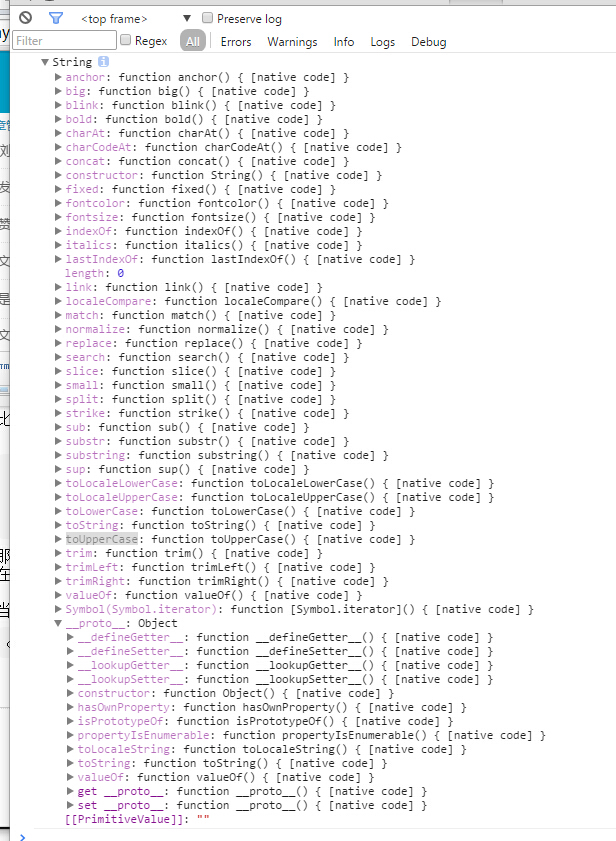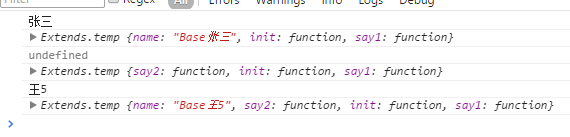# JavaScript高手转正学习(2)-原型的理解

```   var s='1a';
或者

`console.dir(String.prototype);``s.toUpperCase()`

``` String.prototype.sayhello=function(){
}

var s="wj008";
s.sayhello();```

``` //先保存原来的方法为旧的方法 否则 这个方法被覆盖了
String.prototype.OldtoUpperCase  = String.prototype.toUpperCase;
String.prototype.toUpperCase=function(){
}

var s="wj008";
s.toUpperCase();```

``` function Test(){}
var a=new Test();
a.sayhello();

var Test2=Test;
var b=new Test();
b.sayhello();

var Test4;
var Test3=Test4= function(){}
var c=new Test4();
c.sayhello();```

```function Base() {}

Base.prototype.say1 = function () {
};

function Test() {}

Test.prototype.say2 = function () {
};

for (var i in Base.prototype) {
Test.prototype[i] = Base.prototype[i];
}

var a = new Test();
a.say1();
a.say2();

var b = new Base();
b.say1();
b.say2(); //这里是会报错的因为 base 是没有 say2的```

```    function Base() {}

Base.prototype.say1 = function () {
};

function Test() {}

Test.prototype.say2 = function () {
};
var cb=new Base();

for (var i in cb) {
Test.prototype[i] = cb[i];
}

var a = new Test();
a.say1();
a.say2();

var b = new Base();
b.say1();
b.say2(); //这里是会报错的```

```    function Base() {}

Base.prototype.say1 = function () {
};

function Test() {}
Test.prototype=new Base();
Test.prototype.say2 = function () {
};
var a = new Test();
a.say1();
a.say2();

var b = new Base();
b.say1();
b.say2(); //这里是会报错的```

```    function Base() {}

Base.prototype.say1 = function () {
};

function Test() {}
var baseobj=new Base();
baseobj.say2 = function () {
};
Test.prototype=baseobj;
var a = new Test();
a.say1();
a.say2();

var b = new Base();
b.say1();
b.say2(); //这里是会报错的```

```(function(){
function Base() {
this.name='张三';
}

Base.prototype.say1 = function () {
};

function Test() {
this.name='李四';
}
Test.prototype=new Base();
Test.prototype.say2 = function () {
};

function Test2() {}
Test2.prototype=new Test();
Test2.prototype.say2 = function () {
this.name='111';
};

var b1 = new Base();
b1.say1();
console.log(b1);

var a1 = new Test();
a1.say1();
a1.say2();
console.log(a1);

var a2 = new Test2();

a2.say2();
a2.say1();
console.log(a2);
})();```

```(function(){
function Base() {
this.init=function(name){
console.log(name);
this.name='Base'+name;
};
this.init.apply(this,arguments);
}

Base.prototype.say1 = function () {
};

function Test() {
this.init.apply(this,arguments);
}

Test.prototype=new Base();// 构造函数 init 处会输出 undefind
Test.prototype.say2 = function () {
};

function Test2() {
this.init.apply(this,arguments);
}

Test2.prototype=new Test(); // 构造函数 init 处会输出 undefind
Test2.prototype.say2 = function () {
};

var b1 = new Base('张三');
b1.say1();
console.log(b1);

var a1 = new Test('李四');
a1.say1();
a1.say2();
console.log(a1);

var a2 = new Test2('王5');

a2.say2();
a2.say1();
console.log(a2);
})();```

1 判断参数数量 把上面的设置如下。

```(function(){
function Base() {
this.init=function(name){
console.log(name);
this.name='Base'+name;
};
arguments.length==0 || this.init.apply(this,arguments);
}

Base.prototype.say1 = function () {
};

function Test() {
arguments.length==0 ||  this.init.apply(this,arguments);
}

Test.prototype=new Base();
Test.prototype.say2 = function () {
};

function Test2() {
arguments.length==0 ||  this.init.apply(this,arguments);
}

Test2.prototype=new Test();
Test2.prototype.say2 = function () {
};

var b1 = new Base('张三');
b1.say1();
console.log(b1);

var a1 = new Test('李四');
a1.say1();
a1.say2();
console.log(a1);

var a2 = new Test2('王5');

a2.say2();
a2.say1();
console.log(a2);
})();```

2 首参数类型判断

```(function(){
function Base() {
this.init=function(name){
console.log(name);
if(name){
this.name='Base'+name;
}
};
(arguments.length==1 && Base===arguments) || this.init.apply(this,arguments);
}

Base.prototype.say1 = function () {
};

function Test() {
(arguments.length==1 && Test===arguments) ||  this.init.apply(this,arguments);
}

Test.prototype=new Base(Base);
Test.prototype.say2 = function () {
};

function Test2() {
(arguments.length==1 && Test2===arguments) ||  this.init.apply(this,arguments);
}

Test2.prototype=new Test(Test);
Test2.prototype.say2 = function () {
};

var b1 = new Base('张三');
b1.say1();
console.log(b1);

var a1 = new Test();
a1.say1();
a1.say2();
console.log(a1);

var a2 = new Test2('王5');

a2.say2();
a2.say1();
console.log(a2);
})();```

```    (function () {
//继承用的函数
function Extends(func, base) {
var temp = function () {
(arguments.length == 1 && temp === arguments) || this.init.apply(this, arguments);
};
console.log(base);
if (typeof (base) === 'function') {
temp.prototype = new base(base);
}
func.call(temp.prototype);
return temp;
}
//构造Base类
var Base = Extends(function () {
//这里的this 是原型
this.init = function (name) {
console.log(name);
if (name) {
//这里的this 实例对象本身
this.name = 'Base' + name;
}
};
//这里的this 是原型
this.say1 = function () {
};

});
//构造Test 继承Base
var Test = Extends(function () {
//这里的this 是原型
this.say2 = function () {
};
}, Base);

//构造Test2 继承Test
var Test2 = Extends(function () {
this.say2 = function () {
};
}, Test);

var b1 = new Base('张三');
b1.say1();
console.log(b1);

var a1 = new Test();
a1.say1();
a1.say2();
console.log(a1);

var a2 = new Test2('王5');

a2.say2();
a2.say1();
console.log(a2);
})();``````(function () {
//继承用的函数
function Extends(name,func, base) {
eval(' var temp = function '+name+'() {(arguments.length == 1 && temp === arguments) || this.init.apply(this, arguments);};')
if (typeof (base) === 'function') {
temp.prototype = new base(base);
}
func.call(temp.prototype);
return temp;
}
//构造Base类
var Base = Extends('Base',function () {
//这里的this 是原型
this.init = function (name) {
console.log(name);
if (name) {
//这里的this 实例对象本身
this.name = 'Base' + name;
}
};
//这里的this 是原型
this.say1 = function () {
};

});
//构造Test 继承base
var Test = Extends('Test',function () {
//这里的this 是原型
this.say2 = function () {
};
}, Base);

var Test2 = Extends('Test2',function () {
this.say2 = function () {
};
}, Test);

var b1 = new Base('张三');
b1.say1();
console.log(b1);

var a1 = new Test();
a1.say1();
a1.say2();
console.log(a1);

var a2 = new Test2('王5');

a2.say2();
a2.say1();
console.log(a2);
})();```

• 总赞数量：18241

• 总踩数量：128081

• 文章数量：27